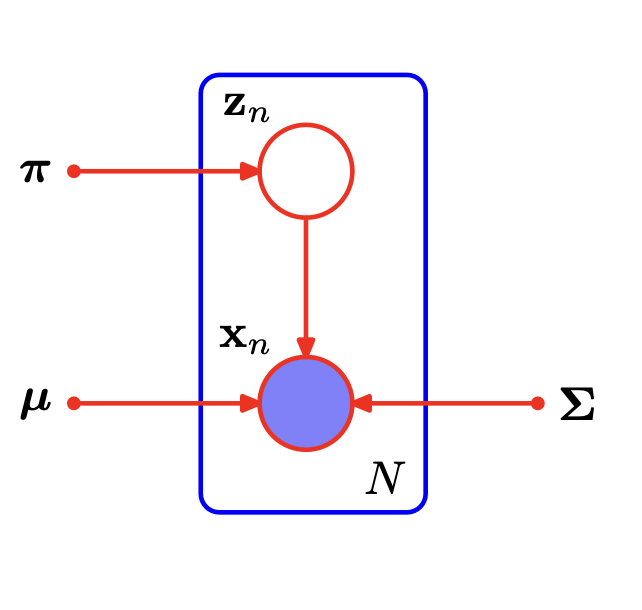# Clustering – Probabilistic Model-Based Methods (2)

4_2_probabilistic_Model_based_methods_2

### 2.1 最大似然$\begin{array}{}\text{(2)}& l\left(\theta \right)=\mathrm{ln}p\left(\mathbf{X}|\pi ,\mu ,\mathbf{\Sigma }\right)=\sum _{n=1}^{N}\mathrm{ln}\left\{\sum _{k=1}^{K}{\pi }_{k}\mathcal{N}\left({\mathbf{x}}_{n}|{\mu }_{k},{\mathbf{\Sigma }}_{k}\right)\right\}\end{array}$

### 2.2 用于高斯混合模型的EM

$\begin{array}{}\text{(3)}& \begin{array}{ccc}\frac{\partial }{\partial {\mu }_{k}}l\left(\theta \right)& =& \sum _{n=1}^{N}\frac{{\pi }_{k}\mathcal{N}\left({\mathbf{x}}_{n}|{\mu }_{k},{\mathbf{\Sigma }}_{k}\right)}{\sum _{j=1}^{K}{\pi }_{j}\mathcal{N}\left({\mathbf{x}}_{n}|{\mu }_{j},{\mathbf{\Sigma }}_{j}\right)}{\mathrm{\Sigma }}_{k}^{-1}\left({\mathbf{x}}_{n}-{\mu }_{k}\right)\\ & =& \sum _{n=1}^{N}\gamma \left({z}_{nk}\right){\mathrm{\Sigma }}_{k}^{-1}\left({\mathbf{x}}_{n}-{\mu }_{k}\right)\end{array}\end{array}$

$\begin{array}{}\text{(4)}& {\mu }_{\mathbf{k}}=\frac{1}{{N}_{k}}\sum _{n=1}^{N}\gamma \left({z}_{nk}\right){\mathbf{x}}_{n}\end{array}$

$\begin{array}{}\text{(5)}& {N}_{k}=\sum _{n=1}^{N}\gamma \left({z}_{nk}\right)\end{array}$

$\begin{array}{}\text{(6)}& {\mathrm{\Sigma }}_{k}=\frac{1}{{N}_{k}}\sum _{n=1}^{N}\gamma \left({z}_{nk}\right)\left({\mathbf{x}}_{n}-{\mu }_{k}\right)\left({\mathbf{x}}_{n}-{\mu }_{k}{\right)}^{T}\end{array}$

$\begin{array}{}\text{(7)}& \mathrm{ln}p\left(\mathbf{X}|\pi ,\mu ,\mathrm{\Sigma }\right)+\lambda \left(\sum _{k=1}^{K}{\pi }_{k}-1\right)\end{array}$

$\begin{array}{}\text{(8)}& \sum _{n=1}^{N}\frac{\mathcal{N}\left({\mathbf{x}}_{n}|{\mu }_{k},{\mathbf{\Sigma }}_{k}\right)}{\sum _{j=1}^{K}{\pi }_{j}\mathcal{N}\left({\mathbf{x}}_{n}|{\mu }_{j},{\mathbf{\Sigma }}_{j}\right)}+\lambda =0\end{array}$

(7)式的第一项相比于(2)少了一个${\pi }_{k}$$\pi_k$,等式两边同时乘${\pi }_{k}$$\pi_k$，可以得到

$\begin{array}{}\text{(9)}& \begin{array}{c}{N}_{k}+{\pi }_{k}\lambda =0\end{array}\end{array}$

$\begin{array}{}\text{(10)}& \lambda =-N\end{array}$

$\begin{array}{}\text{(11)}& {\pi }_{k}=\frac{{N}_{k}}{N}\end{array}$

1. 初始化${\mu }_{k},{\mathrm{\Sigma }}_{k},{\pi }_{k}$$\mu_k,\Sigma_k,\pi_k$,计算对数似然函数(对应本片中的公式(1))的初始值。

2. E步骤。计算当前参数值的"责任":

$\begin{array}{}\text{(12)}& \gamma \left({z}_{nk}\right)=\frac{{\pi }_{k}\mathcal{N}\left(\mathbf{x}|{\mu }_{k},{\mathbf{\Sigma }}_{k}\right)}{\sum _{j=1}^{K}{\pi }_{j}\mathcal{N}\left(\mathbf{x}|{\mu }_{j},{\mathbf{\Sigma }}_{j}\right)}\end{array}$
3. M步骤，重新计算参数:

$\begin{array}{}\text{(13)}& \begin{array}{c}{\mu }_{k}^{new}=\frac{1}{{N}_{k}}\sum _{n=1}^{N}\gamma \left({z}_{nk}\right){\mathbf{x}}_{n}\\ {\mathrm{\Sigma }}_{k}^{new}=\frac{1}{{N}_{k}}\sum _{n=1}^{N}\gamma \left({z}_{nk}\right)\left({\mathbf{x}}_{n}-{\mu }_{k}^{new}\right)\left({\mathbf{x}}_{n}-{\mu }_{k}^{new}{\right)}^{T}\\ {\pi }_{k}^{new}=\frac{{N}_{k}}{N}\end{array}\end{array}$

其中：

$\begin{array}{}\text{(14)}& {N}_{k}=\sum _{n=1}^{N}\gamma \left({z}_{nk}\right)\end{array}$

检查参数或者新的对数似然函数是否收敛，若收敛则结束，否则使用新的参数并返回第2步。